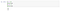# <Python tools> basic calculation & variables

Topic 1: Let’s turn complex financial numbers into easy codes and graphs!

## Python is an easy to learn, powerful programming language. Its efficient high-level data structure helps us efficiently analyze BIG DATA. The following examples are coded with Jupyter Notebook.

1. Using Python as a calculator

2. Output

The print( ) function writes the value of the argument it is given.

The type of “The value of i is” is string, so it required a quote while coding. Strings are printed without quotes, and a space is inserted between items, so you can format things nicely.

3. Assign

While coding, we use = (equals sign) to assign.

a = 10 means that the variable is assigned as 10.

a = a+5 (or a`+=`5) means that the variable is once again assigned as a+5.Caution! This += calculation symbol can not be separated with space.

The calculation symbols `*=``/=``%=``**= `will reassigned to the variable after calculation.

4. Variables

A variable is created when we first assign value to it.

A variable does not need to be declared with any particular type. However, if we want to specify the data type of the variable, this can be done with casting.